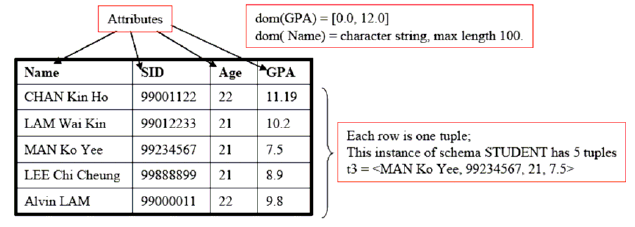FREE E LEARNING PLATFORM

# DBMS Relational Model

We shall represent a relation as a table with columns and rows. Each column of the table has a name, or attribute. Each row is called a tuple.

Relational Model represents how data is stored in Relational Databases. A relational database stores data in the form of relations (tables).

Relational Model was proposed by E.F. Codd to model data in the form of relations or tables.

Domain: a set of atomic values that an attribute can take.

Attribute: name of a column in a particular table (all data is stored in tables). Each attribute Ai must have a domain, dom(Ai).

Relational Schema: The design of one table, containing the name of the table (i.e. the name of the relation), and the names of all the columns, or attributes.

Example: STUDENT( Name, SID, Age, GPA)

Degree of a Relation: the number of attributes in the relation's schema.

Tuple, t, of R( A1, A2, A3,...., An): an ORDERED set of values, < v1, v2, v3,...., vn>, where each vi is a value from dom( Ai).

Relation Instance, r( R): a set of tuples; thus, r( R) = { t1, t2, t3,..., tm}### You may also Find this interesting

DBMS Models

DBMS ER Model

DBMS Relational Model

DBMS Cardinality

RDBMS concepts